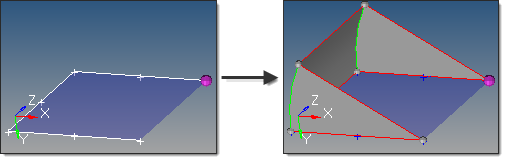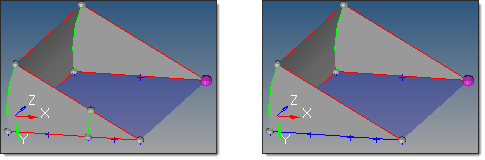﻿ spin subpanel (Surfaces panel)
Manufacturing Solutions

# spin subpanel (Surfaces panel)# spin subpanel (Surfaces panel)This subpanel creates surfaces by spinning lines or a node list around an axis.Seven inputs are required to create a surface using this method:

 • The lines or node list to spin.

If a node list is specified, a line will first be fit through the specified nodes.

 • The merge input lines option applies only for lines.

If disabled, a surface is created for each input line, with shared edges connecting surfaces where relevant.

If enabled, the input lines are merged into smooth lines when possible. A surface is created for each group that forms a tangentially continuous line.The results on the left preserve the two input lines in the foreground; the image on the right merges them.

 • The create in method option applies only for lines.  This defines the resulting surface component organization.

Selecting current component organizes the new surfaces to the current component.

Selecting lines component adds the new surfaces to the same component that the selected lines already belong to.  The result is unpredictable if lines from different components become a part of the same surface.

 • The plane/vector defining the rotation axis.  If a vector is defined or selected, this represents the axis of rotation.  If a plane is defined, the plane normal represents the axis of rotation.

The base node of the plane/vector represents the center of rotation.

 • The start angle defines the initial angle before the lines/nodes are spun.  The angle is measured about the axis of rotation using the right-hand rule.
 • The end angle defines the final angle through which the lines/nodes are spun.  The angle is measured about the axis of rotation using the right-hand rule.  The total angle is given by (end angle - start angle).
 • The direction of the spin.

Spin + is defined using the right-hand rule around the axis of rotation and uses the start angle and end angle values as specified.

Spin - is defined in the opposite direction and uses the negative of the specified start angle and end angle values.

### How do I…

Create surfaces by spinning lines

Create surfaces by spinning nodes